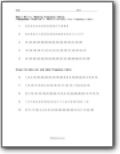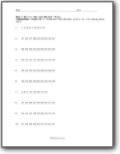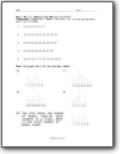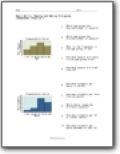### What Are Frequency Tables, Box and Whisker Plots, Line Plots, and Histograms?

These are the terms and practices that we use in mathematics subject to solve different problems. Frequency tables - It is the table of different sections in which we list the items. We can indicate the number of times the items occur. We express the frequency of different products by the English letter (f). Jennifer has to put 6 purple balls in two boxes. He starts dropping each ball in two buckets after the other name X and Y. She drops the ball by a random shot. After dropping 10 balls. After taking a nap, she started repeating the process again. Box and whisker plot - It is a chart or a graph in which we can express information from a five-number frequency. In this table, you can display the distribution of the details like you do with the help of stem and leaf plot or histogram. This table help you in presenting whether you are skewing it or show next unusual observation in the data set. Line plots - It is also a form of graph or table. You can use it to show your data points or check marks above a number line. It will express the frequency of each object’s value. Histogram - It is also a graph that express data with the help of using bars of different heights. Its groups numbers display into ranges.Record the data into frequency table and group the data sets.###### Box and Whisker Plots

Draw box and whisker plots for following data sets.###### Reading and Making Line Plots

Make line plots for following datasets and find the mode.